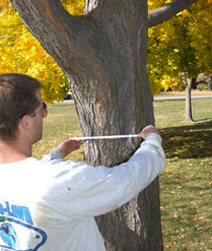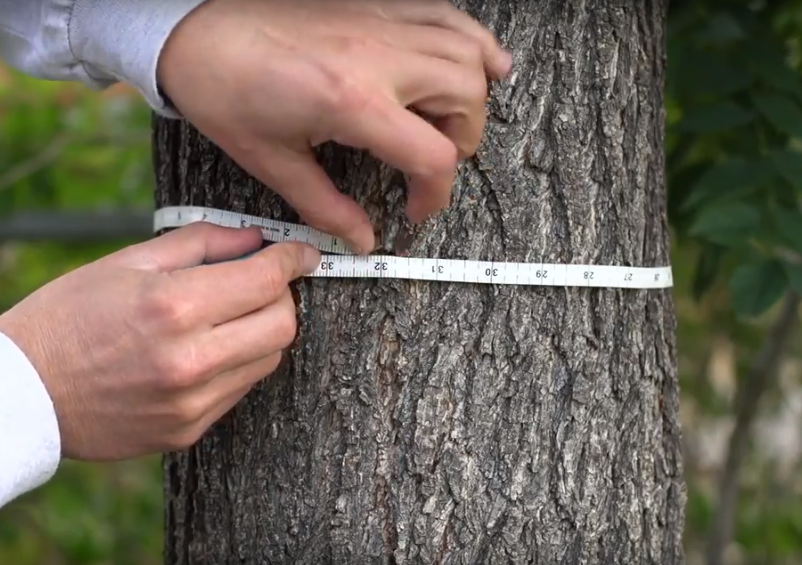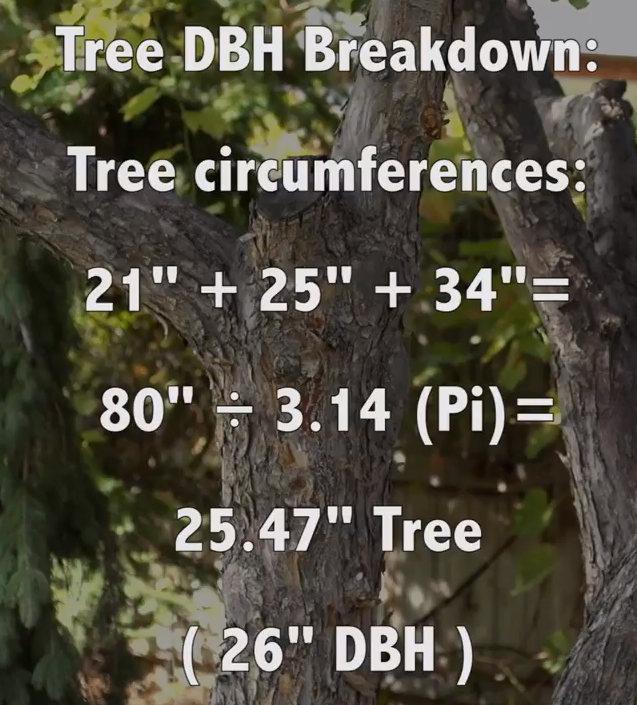# How To Measure The Diameter Breast Height of a Tree## Measuring for a Tree Treatment

To reduce product waste and to give you an accurate price for insect treatment for your tree, we require that you measure the diameter breast height (DBH) of the tree prior to our arrival.
##### Which tree care applications do you need to provide the Diameter Breast Height of a tree?
We need the Diameter Breast Height measurements for the following tree care services: Merit, Orthene, Safari, Tree IV for Chlorosis and Tree-age.

## Quick Measurement Technique

The easiest method to measure the tree DBH is by taking a ruler or a measuring tape and measure the diameter (width) of the tree at chest level (about 4-5 feet from the ground). You will want to round up to the closest inch to make that there is enough product put into the tree to be effective.

## More Accurate Measurement Technique

The slightly more time consuming, but more accurate method is to measure the circumference of the tree by measuring around the tree at chest level and divide by π (pi) or 3.14. This will give you the diameter.
##### Example:
The tree has a 32 inch circumference, measured at about chest height.
• 32 ÷ 3.14 = 10.2”
We will then want to round up to 11” to make sure that the tree is treated with enough product to be effective.## Measuring a Multi-Stem or Clump Tree

To calculate a multi-stem tree you will measure the trunk of each tree and add them together. i.e. For a multi-stem tree with 3 trunks.
##### Step 1 – Measure each trunk:
• Trunk 1 circumference is 21″
• Trunk 2 circumference is 25″
• Trunk 3 circumference is 34″
##### Step 2 – Add each trunk measurement together:
Add together to get the measurement for the total DBH:
• 21 + 25 + 34 = 80″ total diameter
##### Step 3 – Divide total diameter by π (pi) or 3.14:
• 80 ÷ 3.14 = 25.47 (rounded to 26)